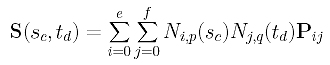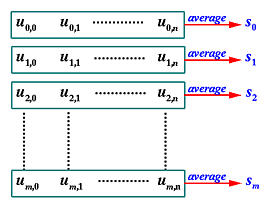# Parameters and Knot Vectors for SurfacesSince a B-spline surface of degree (p,q) defined by e+1 rows and f+1 columns of control points has the following equationit requires two sets of parameters for surface interpolation and approximation. Suppose we have m+1 rows and n+1 columns of data points Dij, where 0 <= i <= m and 0 <= j <= n. Thus, m+1 parameters s0, ..., sm in the u-direction (i.e., one for each row of data points) and n+1 parameters t0, ..., tn in the v-direction (i.e., one for each column of data points) are required so that point (sc,td) in the domain of the surface corresponds to point S(sc,td) on the surface, which, in turn, corresponds to the data point Dcd. Putting this in an equation form, the point on the surface, evaluated at (sc,td) as shown below,corresponds to the data point Dcd, where sc and td are parameters in the u- and v-direction, respectively.

In the equation of the desired B-spline surface, the u-direction corresponds to the index i in Ni,p(u) and Pij. Since i runs from 0 to m, N0,p(u), N1,p(u), ..., Nm,p(u) are coefficients of the columns of the control points. Therefore, in the u-direction, we need m+1 parameters, and using a parameter computation method that takes degree p and the data points on column j, we can compute m+1 parameters u0,j, u1,j, ..., um,j. This is shown in the figure below. The desired parameters s0, s1, ..., sm are simply the average of each row. More precisely, parameter si is the average of parameters on row i, si = (ui,0 + ui,1 + ... + ui,n)/(n+1).The computation of parameters for the v-direction is similar. Each row of data points has n+1 points and hence requires n+1 parameters. Thus, for data points on row i, we can compute n+1 parameter values vi,0, vi,1, ..., vi,n. Since we have m+1 rows, these values can be organized into a (m+1)×(n+1) matrix, and parameter tj is simply the average of the parameters on column j, tj = (v0,j + v1,j + ... + vm,j)/(m+1). In this way, we obtain n+1 parameters for the v-direction.

This procedure is summarized as follows:

 Input: (m+1)×(n+1) data points Di,j; Output: Parameters in the u-direction s0, ..., sm           and parameters in the v-direction t0, ..., tn; Algorithm: /* calculate s0, ..., sm */ for j := 0 to n do /* column j */ Compute a set of m+1 parameters u0,j, u1,j, ..., um,j; for i := 0 to m do si = (ui,0 + ui,1 + ... + ui,n)/(n+1); /* parameters for the u-direction are available */ /* calculate t0, ..., tn */ for i := 0 to m do /* row i */ Compute a set of n+1 parameters vi,0, vi,1, ..., vi,n; for j := 0 to n do tj = (v0,j + v1,j + ... + vn,j)/(m+1); /* parameters for the v-direction are available */

Then, parameter values s0, s1, ..., sm and degree p jointly define a knot vector U in the u-direction. Similarly, parameters t0, t1, ..., tn and degree q jointly define a knot vector V in the v-direction. Click here to review the way of computing a knot vector from a set of parameters.

Note that the above is only a conceptual algorithm and is inefficient. Once you know what is going on, it is quite easy to convert it to a very efficient algorithm. Note also that this algorithm only works for the uniformly spaced, chord length and centripetal methods. For the universal method, because there is no data points involved, we can apply uniform knots to one row and one column of data points for computing the parameters.